KCC2.2
 Name:    KCC2.2

Multiple Choice
Identify the choice that best completes the statement or answers the question.

1.

Which number does not fit in the sequence below?
2, 4, 5, 8, 10
 a. 8 d. 5 b. 10 e. 4 c. 2

2.

Which of the numbers below is not in the correct sequence?
3 ,6 ,10 ,12
 a. 6 c. 3 b. 10 d. 12

3.

Which number does not belong in the following sequence?
1, 2, 6, 4, 5
 a. 6 d. 1 b. 5 e. 2 c. 4

4.

Which picture would come next?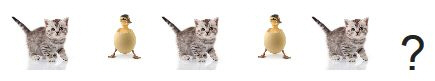a.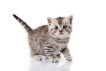b.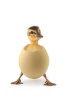5.

Which animal would come next?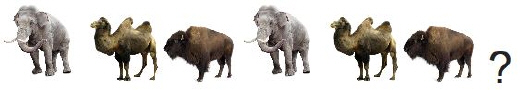a.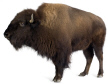c.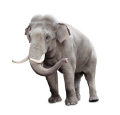b.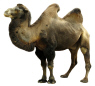6.

Which animal would come next?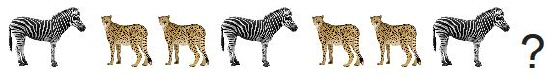a.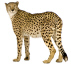b.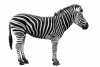7.

Which bunny would come next?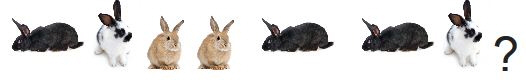a.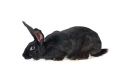c.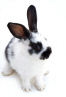b.8.

Which dinosaur comes next?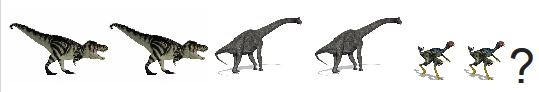a.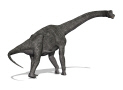c.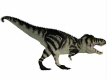b.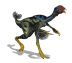9.

Which snake would come next?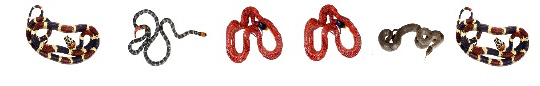a.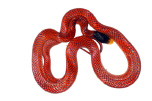c.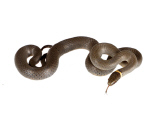b.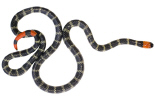d.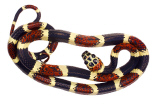10.

Which number comes next in the pattern?
1, 1, 2, 2, 4, 1, 1, 2, ?
 a. 1 c. 4 b. 2 d. 3